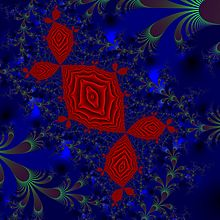Douady rabbit in an exponential family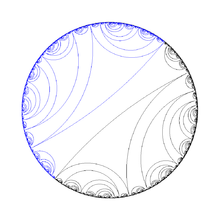Lamination of rabbit Julia setQuaternion julia set with parameters c = −0,123 + 0.745i and with a cross-section in the XY plane. The "Douady Rabbit" julia set is visible in the cross section

The Douady rabbit, named for the French mathematician Adrien Douady, is any of various particular filled Julia sets associated with the c near the center period 3 buds of Mandelbrot set for complex quadratic map.

## Forms of the complex quadratic map

There are two common forms for the complex quadratic map$\mathcal M$. The first, also called the complex logistic map, is written as$z_{n+1} = \mathcal M z_n = \gamma z_n \left(1 - z_n\right),$

where z is a complex variable and γ is a complex parameter. The second common form is$w_{n+1} = \mathcal M w_n = w_n^2 - \mu.$

Here w is a complex variable and μ is a complex parameter. The variables z and w are related by the equation$z = -\frac{w}{\gamma}+\frac{1}{2},$

and the parameters γ and μ are related by the equations$\mu = \left(\frac{\gamma-1}{2}\right)^2 - \frac{1}{4} \quad,\quad \gamma = 1 \pm \sqrt{1 + 4\mu}.$

Note that μ is invariant under the substitution$\gamma \to 2 - \gamma$.

## Mandelbrot and filled Julia sets

There are two planes associated with$\mathcal M$. One of these, the z (or w) plane, will be called the mapping plane, since$\mathcal M$ sends this plane into itself. The other, the γ (or μ) plane, will be called the control plane.

The nature of what happens in the mapping plane under repeated application of$\mathcal M$ depends on where γ (or μ) is in the control plane. The filled Julia set consists of all points in the mapping plane whose images remain bounded under indefinitely repeated applications of$\mathcal M$. The Mandelbrot set consists of those points in the control plane such that the associated filled Julia set in the mapping plane is connected.

Figure 1 shows the Mandelbrot set when γ is the control parameter, and Figure 2 shows the Mandelbrot set when μ is the control parameter. Since z and w are affine transformations of one another (a linear transformation plus a translation), the filled Julia sets look much the same in either the z or w planes.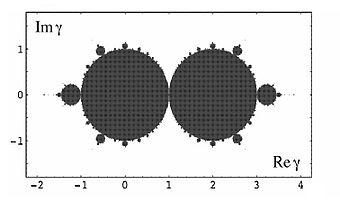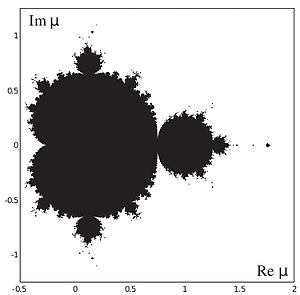Figure 1: The Mandelbrot set in the γ plane. Figure 2: The Mandelbrot set in the μ plane.

The Douady rabbit is most easily described in terms of the Mandelbrot set as shown in Figure 1. In this figure, the Mandelbrot set, at least when viewed from a distance, appears as two back-to-back unit discs with sprouts. Consider the sprouts at the one- and five-o'clock positions on the right disk or the sprouts at the seven- and eleven-o'clock positions on the left disk. When γ is within one of these four sprouts, the associated filled Julia set in the mapping plane is a Douady rabbit. For these values of γ, it can be shown that$\mathcal M$ has z = 0 and one other point as unstable (repelling) fixed points, and$z=\infty$ as an attracting fixed point. Moreover, the map${\mathcal{M}}^3$ has three attracting fixed points. Douady's rabbit consists of the three attracting fixed points z1, z2, and z3 and their basins of attraction.

For example, Figure 3 shows Douady's rabbit in the z plane when γ = γD = 2.55268 − 0.959456i, a point in the five-o'clock sprout of the right disk. For this value of γ, the map$\mathcal M$ has the repelling fixed points z = 0 and z = .656747 − .129015i. The three attracting fixed points of${\mathcal M}^3$ (also called period-three fixed points) have the locations$z^1 = 0.499997032420304 - (1.221880225696050\times10^{-6})i{\;}{\;}{\mathrm {(red)}},$$z^2 = 0.638169999974373 - (0.239864000011495)i{\;}{\;}{\mathrm {(green)}},$$z^3 = 0.799901291393262 - (0.107547238170383)i{\;}{\;}{\mathrm {(yellow)}}.$

The red, green, and yellow points lie in the basins B(z1), B(z2), and B(z3) of${\mathcal M}^3$, respectively. The white points lie in the basin$B(\infty)$ of$\mathcal M$.

The action of$\mathcal M$ on these fixed points is given by the relations${\mathcal M}z^1=z^2,$${\mathcal M}z^2=z^3,$${\mathcal M}z^3=z^1.$

Corresponding to these relations there are the results${\mathcal M}B(z^1)=B(z^2) {\;} {\mathrm {or}} {\;} {\mathcal M}{\;}{\mathrm {red}}\subseteq{\mathrm {green}},$${\mathcal M}B(z^2)=B(z^3) {\;} {\mathrm {or}} {\;} {\mathcal M}{\;}{\mathrm {green}}\subseteq{\mathrm {yellow}},$${\mathcal M}B(z^3)=B(z^1) {\;} {\mathrm {or}} {\;} {\mathcal M}{\;}{\mathrm {yellow}}\subseteq{\mathrm {red}}.$

Note the marvelous fractal structure at the basin boundaries.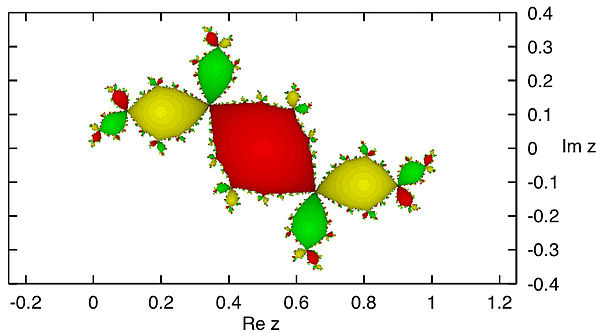Figure 3: Douady's rabbit for γ = 2.55268 − 0.959456i or μ = 0.122565 − 0.744864i.

As a second example, Figure 4 shows a Douady rabbit when γ = 2 − γD = − .55268 + .959456i, a point in the eleven-o'clock sprout on the left disk. (As noted earlier, μ is invariant under this transformation.) The rabbit now sits more symmetrically on the page. The period-three fixed points are located at$z^1= 0.500003730675024 + (6.968273875812428 \times 10^{-6})i {\;}{\;} ({\mathrm {red}}),$$z^2=-0.138169999969259 + (0.239864000061970)i {\;}{\;} ({\mathrm {green}}),$$z^3= -0.238618870661709 - (0.264884797354373)i {\;}{\;} ({\mathrm {yellow}}),$

The repelling fixed points of$\mathcal M$ itself are located at z = 0 and z = 1.450795 + 0.7825835i. The three major lobes on the left, which contain the period-three fixed points z1,z2, and z3, meet at the fixed point z = 0, and their counterparts on the right meet at the point z = 1. It can be shown that the effect of$\mathcal M$ on points near the origin consists of a counterclockwise rotation about the origin of arg(γ), or very nearly$120^\circ$, followed by scaling (dilation) by a factor of | γ | = 1.1072538.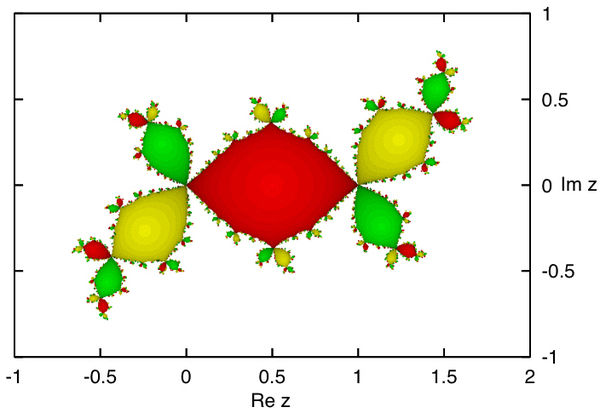Figure 4: Douady's rabbit for γ = − 0.55268 + 0.959456i or μ = 0.122565 − 0.744864i.

Wikimedia Foundation. 2010.

### См. также в других словарях:

• Dynamique holomorphe — La dynamique holomorphe est un domaine de l analyse complexe et des systèmes dynamiques s intéressant principalement à l étude de l itération des applications holomorphes. Sommaire 1 Historique 2 Dynamique holomorphe à une variable 2.1… …   Wikipédia en Français

• Liste De Fractales Par Dimension De Hausdorff — Cet article est une liste de fractales, ordonnées par dimension de Hausdorff croissante. En mathématiques, une fractale est un ensemble dont la dimension de Hausdorff (notée δ) est strictement supérieure à la dimension topologique. Sommaire 1… …   Wikipédia en Français

• Liste de fractales — par dimension de Hausdorff Cet article est une liste de fractales, ordonnées par dimension de Hausdorff croissante. En mathématiques, une fractale est un ensemble dont la dimension de Hausdorff (notée δ) est strictement supérieure à la dimension… …   Wikipédia en Français

• Liste de fractales par dimension de Hausdorff — Cet article est une liste de fractales, ordonnées par dimension de Hausdorff croissante. En mathématiques, une fractale est un ensemble dont la dimension de Hausdorff (notée δ) est strictement supérieure à la dimension topologique. Sommaire 1… …   Wikipédia en Français

• Liste de fractales par dimension de hausdorff — Cet article est une liste de fractales, ordonnées par dimension de Hausdorff croissante. En mathématiques, une fractale est un ensemble dont la dimension de Hausdorff (notée δ) est strictement supérieure à la dimension topologique. Sommaire 1… …   Wikipédia en Français

• List of mathematics articles (D) — NOTOC D D distribution D module D D Agostino s K squared test D Alembert Euler condition D Alembert operator D Alembert s formula D Alembert s paradox D Alembert s principle Dagger category Dagger compact category Dagger symmetric monoidal… …   Wikipedia

• Fibonacci number — A tiling with squares whose sides are successive Fibonacci numbers in length …   Wikipedia

• Glires — Taxobox name = Glires fossil range = Early Paleocene Recent image width = 250px image caption = Kangaroo rat ( Dipodomys ) regnum = Animalia classis = Mammalia infraclassis = Eutheria superordo = Euarchontoglires unranked familia = Glires… …   Wikipedia

### Поделиться ссылкой на выделенное

##### Прямая ссылка:
Нажмите правой клавишей мыши и выберите «Копировать ссылку»